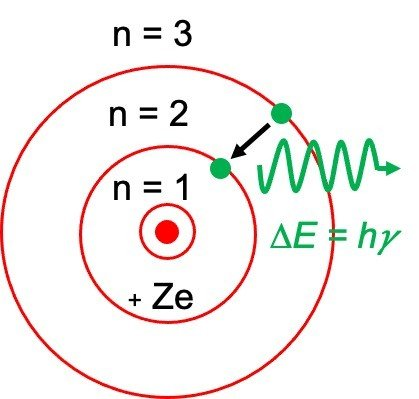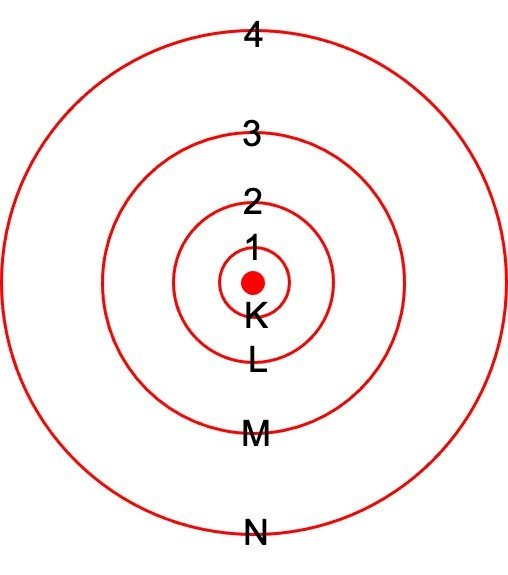Research Tweet

# What is Bohr Model?

o The Bohr model was an improved form of earlier cubic model (1902), the plum-pudding model (1904), the Saturnian model (1904), and the Rutherford model.

o The Bohr model is sometimes also referred to as the Rutherford-Bohr model.

o Bohr combined Planck’s and Einstein’s quantization ideas into a model of the hydrogen atom that set the contradiction of atom stability and discrete spectra.

o The Bohr model of the hydrogen atom elucidates the connection between the quantization of photons and the quantized emission from the atoms.

o Bohr described the hydrogen atom in terms of an electron that moves in a circular orbit around a nucleus.

o He hypothesized that the electron was thus limited to certain orbits termed as discrete energy levels.

o Electrons can also jump between these orbits either by absorbing or releasing photons.

o When an electron travels from a higher-energy orbit to a more stable orbit, energy is emitted in the form of a photon. And when an electron jumps from a stable orbit to a more excited orbit, a photon of energy is absorbed.

o Hence, by using the Bohr model, we can analyse the energy of an electron and the radius of its orbit in any one-electron system.

## Characteristic of Bohr Model

o The Bohr model represents that the electrons present in any atom revolve in orbits of different energy around the nucleus just like planet revolve around the sun.

o The term energy levels (or shells) are used to define these orbits of differing energy. He stated that the energy of an electron is quantized, which means electrons can move from one energy level to another but there is nothing in between.

o The energy level that is occupied by an electron is called its ground state. But by absorbing energy it can thus move to a higher-energy, less-stable level, or shell. This higher-energy, or less-stable state is termed as excited state of the electron.

o The electron can also return to its original, stable ground state by releasing the energy it has absorbed, as shown in the figure below.

### Bohr Model of Atom###### Ground and Excited States of Electron

o Bohr stated that an electron needs less energy if it close to the nucleus, but as it moves away from nucleus it needs more amount of energy.

o The frequency of the photon of light thus emitted depends upon the variance of two energy levels.

E2-E1 = hv

Where E2 is defined as the energy of higher level and E1 is defined as the energy of lower level and h is termed as Planck’s Constant.

o So, Bohr numbered the different energy levels or shells. These are numbered as 1,2,3,4…or K, L, M, N…. shells preliminary from the shell near to nucleus.

o Bohr also found that the numerous energy levels can occupy only a fixed number of electrons for instance; energy level 1 or K shell can accommodate only 2 electrons, energy level 2 or L shell has 8 electrons, and so on.

o Number in various shell is determined by the formula – 2n2 where n is defined as number of shells assigned to various energy shell of an atom.

#### Bohr Model of Atom##### Merits of Bohr model

o The Bohr model was the foremost model to postulate the quantization of electron orbits in atoms. Thus, it indicates an early quantum theory that also gave a way to development of modern quantum theory. It presented the concept of a quantum number to define the atomic states of any atom.

o Bohr described the stability of atoms. He stated that an electron does not radiate energy if it is present in the same shell.

o He explained the spectrum of hydrogen and he also calculated the size of atom was accurately. The drawback of Rutherford model of atom was that the atoms become unstable when accelerated charge, radiated energy, and lastly fell into nucleus. This theory of Rutherford was thus eliminated.

###### Limitations of Bohr Model

o The Bohr atomic model theory explains that electrons of any atom have both a known radius and an orbit that is, fixed position and momentum at the same time, which is nearly impossible according to Heisenberg Uncertainty Principle.

o The Bohr atomic model theory properly explained the structure of small sized atoms for instance – hydrogen, but for larger atoms poor spectral predictions are obtained. Hence, it could not explain the structure of large sized atoms.

o The Zeeman effect could not be explained by Bohr which states that the spectral line generally splits into several components in the presence of a magnetic field.

o The Stark effect could not be explained by Bohr, which states that the spectral line generally splits into fine lines in the presence of an electric field.

o Bohr model cannot predict the relative intensities of several spectral lines.

Share

###### Similar Post: